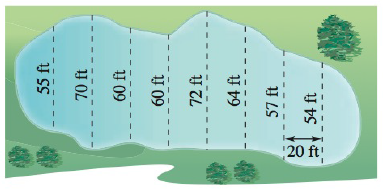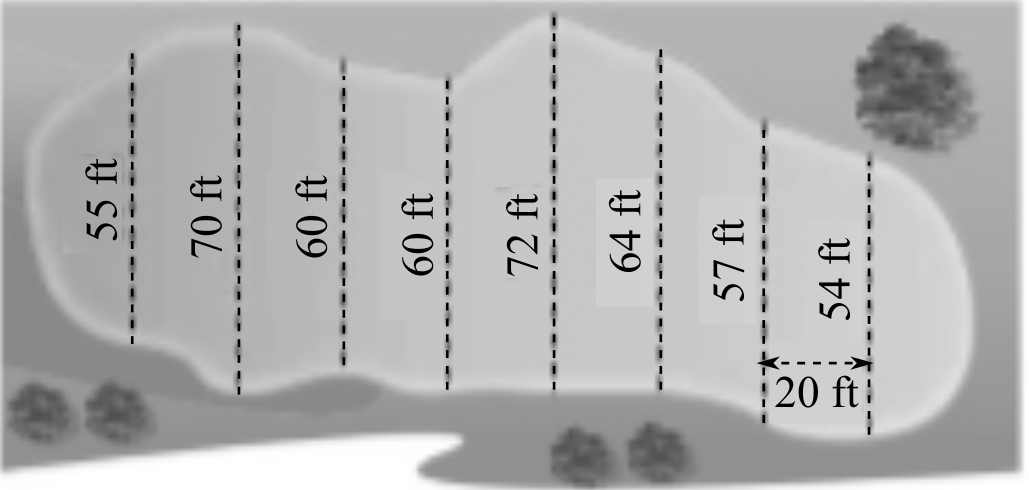Chapter 5, Problem 115RE### Calculus: An Applied Approach (Min...

10th Edition
Ron Larson
ISBN: 9781305860919

#### Solutions

Chapter
Section### Calculus: An Applied Approach (Min...

10th Edition
Ron Larson
ISBN: 9781305860919
Textbook Problem
1 views

# Surface Area Use the Midpoint Rule to estimate the surface area of the swamp shown in the figure.To determine

To calculate: The surface area of the swamp shown below in the figure:Explanation

Given information:

The provided figure is shown below:

Formula used:

Steps to solve a definite integral abf(x)dx with the help of midpoint rule.

Step 1: For a given interval [a,b] divide it into n subintervals with having width of,

Δx=ban

Step 2: Evaluate the midpoint for the given subinterval. Midpoints={x1,x2,x3,xn}

Step 3: Find the value of f at each midpoint and make the sum as shown below:

abf(x)dxban[f(x1)+f(x2)+f(x3)++f(xn)]

Fundamental theorem of calculus:

abf(x)dx=F(x)]ab=F(b)F(a)

Calculation:

Consider the figure.

The lake is divided into 9 parts and the width of each part is 20 ft therefore the total width is:

55+70+60+60+72+64+57+54=180

Thus, Δx=180 and n=9

### Still sussing out bartleby?

Check out a sample textbook solution.

See a sample solution

#### The Solution to Your Study Problems

Bartleby provides explanations to thousands of textbook problems written by our experts, many with advanced degrees!

Get Started